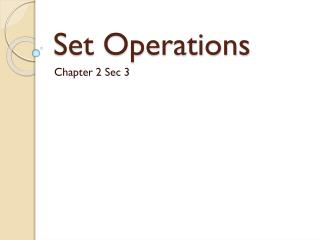DownloadDownload PresentationSet Operations

# Set Operations

Download Presentation## Set Operations

- - - - - - - - - - - - - - - - - - - - - - - - - - - E N D - - - - - - - - - - - - - - - - - - - - - - - - - - -
##### Presentation Transcript

1. Set Operations Chapter 2 Sec 3

2. Union • What does the word mean to you? • What does it mean in mathematics?

3. Definition • The union of sets A and B, written , is the set of elements that are members of either A or B(or both). • Using set-builder notation, {x:x is a member of A or x is a member of B}.

4. The union of more than two sets is the set of all elements belonging to at least one of the sets.

5. Example • Find the union of the following pair of sets. • A={1,3,5,8,9} and B={2,4,6,7,8,}

6. Solution • = {1,2,3,4,…,9} • The solution in the final answer we list the elements in order and did not list duplicate elements because doing so does not affect set equality.

7. Intersection • The essential idea of intersection is a region that is common to both sets.

8. Definition • The intersection of sets A and B, written , is the set of elements common to both A and B. • Using set-builder notation, ={x:x is a member of A and x is a member of B}.

9. The intersection of more than two sets is the set of elements that belong to each of the sets. • If =, then we say that A and B are disjoint.

10. r3 Inside the 2 circles are shaded.

11. r3 Which region would be shaded?

12. Complement • If A is a subset of the universal set U, the complement of A is the set of elements of U that are not elements of A. This set is denoted by A’. • Using set builder notation,

13. Venn diagram: Complement r1 What would be the complement?

14. Example • Find the complement of each set. • U ={1,2,3,…10} and A ={2,4,6,8} • A’ = {1,3,5,7,9} • U is the set of cards in a standard 52 card deck, and F is the set of face cards. • F’ is the set of nonface cards.

15. Set difference • The difference of sets B and A is the set of elements that are in B but not in A. This set is denoted by B-A. • Using set builder notation, • B-A = {x:x is a member of B and x is not a member of A}.

16. Venn for set difference, B-A B A r3 Which region would be shaded?

17. Examples of Set difference. • Find the set difference. • Find {3, 6, 9, 12}-{x:x is an even integer} • Therefore we will remove all the even integers to get {3, 9}